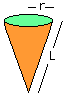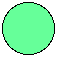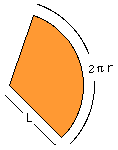Date: Thu, 24 Apr 1997 12:34:29 -0400
Sender: Gary

Subject: Formulae for surface area

Hi, kind folks.

I'm trying to help my son with his Math homework (Grade 10) and he has to find the surface area of a cone and rectangluar pyramid. We have the formulas for the volume of these solids, but can't seem to locate the formulas for surface area.

Can anyone help me out or point me to a source for such formulas?

Many thanks!

Cheers,
Gary

Hi Gary,

One way to find surface area of a three dimensional figure is, if possible, to cut it open and lay it out in the plane. Measure the areas of the pieces in the plane and add those up:
e.g. the 1x1x1 cube - layed out is six 1x1 squares - area 6.For the cone - the area will depend on the angle of the cone. Given a cone of side length L, and top - a circle of radius r, we have two pieces:(a) the top circle pi r2(b) the side - which slit open is a sector of a circle of radius L. However it is a fraction of this circle, the fraction is 2pr over 2pi L (the ratio of the circumference of a whole circle to the circumference of the sector). Hence the area of the side piece is pi L2(r/L) = pi Lr.

For the rectangular pyramid: this can be slit open and seen as a rectangle plus four triangles. Depending on the information given, it may be easy to get the areas of the triangle, or it may require some trigonometry.

Go to Math Central